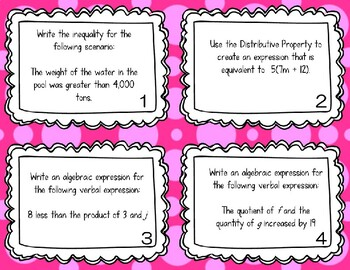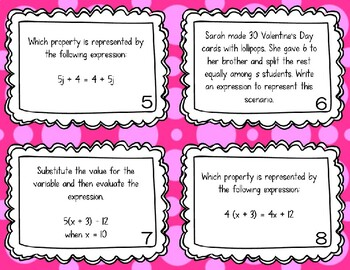# TASK CARDS - Eureka Module 4 - Expressions, Equations, InequalitiesSubject
Resource Type
File Type

Presentation (Powerpoint) File

(1 MB|9 pages)
Standards
• Product Description
• StandardsNEW

24 Engaging Task Cards covering everything from Module 4 in the Eureka Math Curriculum for 6th Grade. This is a collection of mixed review problems, including the following:

- Exponents

- Order of Operations

- Expressions: Writing and Evaluating

- One-Step Equations

- Inequalities: Writing, Solving, and Graphing

Identify when two expressions are equivalent (i.e., when the two expressions name the same number regardless of which value is substituted into them). For example, the expressions 𝘺 + 𝘺 + 𝘺 and 3𝘺 are equivalent because they name the same number regardless of which number 𝘺 stands for.
Apply the properties of operations to generate equivalent expressions. For example, apply the distributive property to the expression 3 (2 + 𝘹) to produce the equivalent expression 6 + 3𝘹; apply the distributive property to the expression 24𝘹 + 18𝘺 to produce the equivalent expression 6 (4𝘹 + 3𝘺); apply properties of operations to 𝘺 + 𝘺 + 𝘺 to produce the equivalent expression 3𝘺.
Evaluate expressions at specific values of their variables. Include expressions that arise from formulas used in real-world problems. Perform arithmetic operations, including those involving whole-number exponents, in the conventional order when there are no parentheses to specify a particular order (Order of Operations). For example, use the formulas V = s³ and A = 6 s² to find the volume and surface area of a cube with sides of length s = 1/2.
Identify parts of an expression using mathematical terms (sum, term, product, factor, quotient, coefficient); view one or more parts of an expression as a single entity. For example, describe the expression 2 (8 + 7) as a product of two factors; view (8 + 7) as both a single entity and a sum of two terms.
Write expressions that record operations with numbers and with letters standing for numbers. For example, express the calculation “Subtract 𝘺 from 5” as 5 - 𝘺.
Total Pages
9 pages
Included
Teaching Duration
N/A
Report this Resource to TpT
Reported resources will be reviewed by our team. Report this resource to let us know if this resource violates TpT’s content guidelines.